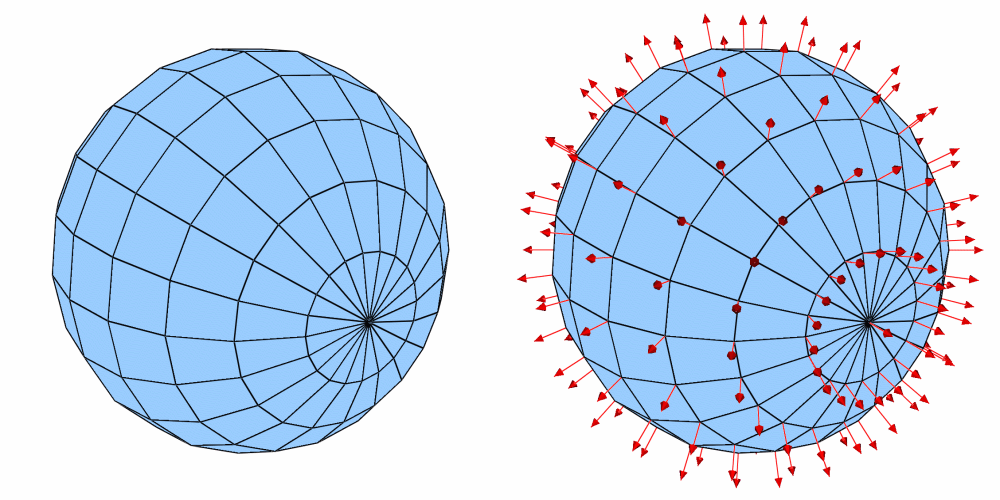# 4.3.8. DeferExpression operator¶

The DeferExpression operator is a special-purpose operator that defers expression execution until later in VisIt’s pipeline execution cycle. This means that instead of expression evaluation taking place before any operators are applied, expression evaluation can instead take place after operators have been applied, at whatever point in the pipeline the DeferOperator exists. This may be necessary in cases where an expression involves the output of an operator, or the operator behaves in such a way as to change the outcome of an expression.

## 4.3.8.1. Plotting surface normals¶

VisIt can use the DeferExpression operator in conjunction with the ExternalSurface operator and the surface_normal expression to plot surface normals for your plot geometry. To plot surface normals, first create a vector expression using the surface_normal expression , which takes the name of your plot’s mesh as an input argument. Once you have done that, you can create a Vector plot of the new expression. Be sure to apply the ExternalSurface operator first to convert the plot’s 2D cells or 3D cells into polygonal geometry that can be used in the surface_normal expression. Finally, apply the DeferExpression operator and set its variable to your new vector expression. This will ensure that the surface_normal expression is not evaluated until after the ExternalSurface operator has been applied.Fig. 4.29 DeferExpression operator example

## 4.3.8.2. Deferring multiple expressions¶

What if you want to color a surface by a new variable equal to (1.0 - X)^2 where X is the x-component of the surface’s normal? Starting with the previous example, and supposing the surface normal expression was defined as surfn=point_surface_normal("mesh"). You would create a new expression to grab the x-component of the normal: X=(1.0-surfn)^2. Add a Pseudocolor plot of X. Apply the ExternalSurface operator. Apply the DeferExpression operator and add both surfn and X to the list of variables being deferred.